Home / Blog / 6 algorithms every developer should know# 6 algorithms every developer should know

•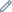Anna Kosheva
•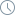July 31, 2022

Let’s talk about the basic knowledge of developers, namely algorithms. What algorithms are worth knowing? Here is a selection of six of the most important.

1. Binary search algorithm

Binary Search is a searching algorithm for finding an element’s position in a sorted array. In this approach, the element is always searched in the middle of a portion of an array.

Binary Search Algorithm can be implemented in two ways which are discussed below.

1. Iterative Method
1. Recursive Method

BFS follows the “go wide, bird’s eye-view” concept. Instead of moving along a certain path to the end, BFS involves moving forward one neighbor at a time.

Instead of following a path, BFS involves visiting the nearest neighbors to s in one step, then visiting the neighbors’ neighbors, and so on until t is found.

How do you know which neighbors to visit first?

To do this, we can use the concept of “first in, first out” (first-in-first-out, FIFO) from the queue (queue). We enqueue first the vertex closest to us, then its unvisited neighbors, and continue this process until the queue is empty or until we find the desired vertex.

1. Depth-first search algorithm

DFS follows the “go deep, head first” concept. The idea is that we move from the starting vertex (point, place) in a certain direction (along a certain path) until we reach the end of the path or destination (the desired vertex). If we have reached the end of the path, but it is not the destination, then we return (to the point of fork or divergence of the paths) and follow a different route.

We are moving along a certain path to the end. If the end of the path is the desired vertex, we’re done. If not, we go back and move along a different path until we explore all the options.

1. Merge sort algorithm

Merge Sort is one of the most popular sorting algorithms that is based on the principle of the Divide and Conquer Algorithms.

Our task is to merge two subarrays A[p..q] and A[q+1..r] to create a sorted array A[p..r]. So the inputs to the function are A, p, q and r

The merge function works as follows:

1. Create duplicates of the subarrays L ← A[p..q] and M ← A[q+1..r].
2. Create three pointers “i”, “j” and “k”
1. “i” maintains a current index of L, starting at 1
2. “j” maintains a current index of M, starting at 1
3. “k” maintains the current index of A[p..q], starting at “p”.
3. Until we reach the end of either “L” or “M”, pick the larger among the elements from “L” and “M” and place them in the correct position at A[p..q]
4. When we run out of elements in either “L” or “M”, pick up the remaining elements and put them in A[p..q]
1. Kruskal’s algorithm

Kruskal’s algorithm is a minimum spanning tree algorithm that takes a graph as input and finds the subset of the edges of that graph which

• form a tree that includes every vertex
• has the minimum sum of weights among all the trees that can be formed from the graph

The steps for implementing Kruskal’s algorithm are as follows:

1. Sort all the edges from low weight to high
2. Take the edge with the lowest weight and add it to the spanning tree. If adding the edge created a cycle, then reject this edge.
3. Keep adding edges until we reach all vertices.

Leran more: How to config providers?

1. Tree traversal algorithm

Traversing a tree means visiting every node in the tree. You might, for instance, want to add all the values in the tree or find the largest one. For all these operations, you will need to visit each node of the tree.

Let’s think about how we can read the elements of the tree in the image shown above.

Starting from top, left to right

1 -> 12 -> 5 -> 6 -> 9

Starting from bottom, left to right

5 -> 6 -> 12 -> 9 -> 1

•Anna Kosheva
•July 31, 2022

##### If you like this blog post, you may want to watch
Start: March 28, 2021
Intermediate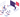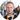Max Pronko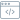20 lessons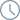15 hours
Adobe Certified Professional — Magento Commerce Developer Study Group is an 10-week (1.5 hours/week) instructor-led study group. This study group will help PHP and JavaScript developers evaluate their readiness and prepare for the Adobe Certified Professional — Magento Commerce Developer exam.
Start: March 15, 202180 lessons15 hours